Digital signal processing involves many different operations. Although there are some algorithms with a high level of digitalization, they can be computed only with additions and shifts, others require specific hardware. For example, we need multiplicators to use an IIR filter, since the input and the output must be multiplied by the coefficients. Fortunately, microprocessors and FPGA includes multiplicators. We can find specific instructions in all processors architecture (ARM multiplicator, RISC-V mutiplicator), and also, in FPGA we can find a significant number of multipliers, that are essentially, hardware structures capable to perform multiplications, and generally, also additions.

That’s great! we can perform additions (also subtractions changing the sign of the operands), and multiplications, we can do everything!… or not? Lets go with an example. We have to implement an speed control for a DC motor. To read the speed of the motor, we have to read the signal of an encoder. The output of the encoder is a pulse train, so to calculate the speed, we can calculate the frequency of the pulses by measuring the time between positive edges. Frequency can be calculated as follows.

$f = \frac{1}{\delta t}$

Then the result can be multiply by the amount of degrees between edges, which is a mechanical parameter, and then we can obtain the speed. Notice that, in this case, we have to perform a multiplication by a reciprocal of a variable, in other words, we have to perform a division. Since there is no specific hardware to perform a division, like we can find to perform additions and multiplications, that means that we have to develop an HDL code to implement an algorithm able to divide two numbers, so, lets go.

In order to perform a division in a digital system, exist some algorithms. In this post I will implement three of them that only involves digital operations like shifts and additions.

First of all, we are going to remember how we can calculate the quotient of a division manually. If we have the operation 5160 divided by 4, as we learned in the school, first we have to find a number that multiplied by four will be near than the most significant digit of the numerator, but always have to be less, for example, a number multiplied by four that has a result near to five, but less than five is 1. Then, we have to calculate the difference between the result of that multiplication, and the digit of the dividend, in this case, one. Next, we will lower the next digit of the dividend, and repeat the operation. If the resulting number, after lower the next digit is less than four, then the number multiplied by the divisor will be zero. Finally, when all the digits of the numerator are used, we will obtain the quotient, and also the remainder.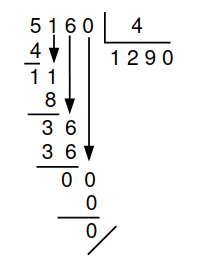Can we do the same with binary numbers? the answer is yes, and that algorithm is named long division. The process is the same as we did for decimal numbers, but this time, the only digits that we can add to the quotient are one and zero. If the value below the numerator is greater than the denominator, then we will add a one in the quotient, on the other hand, if the value is less than the denominator, we will add a zero in the quotient, and we have to lower the next value until the subtraction by the denominator will be positive.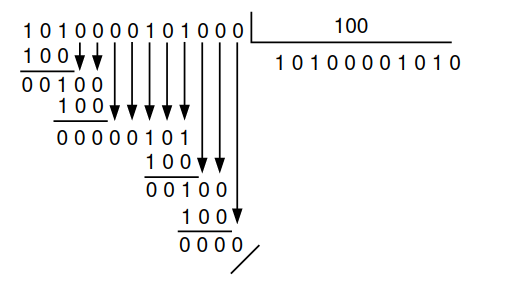The translation of the long division algorithm to an HDL code can be found in the following code. The entire algorithm has been implemented using a finite state machine (FSM), with 4 states. The state zero is used to register the input values of the numerator and denominator. making this we will be tolerant to the top module could change the values in the middle of the process. Also, in this state we will ensure that the value of the denominator will be greater than zero. In other case, the algorithm will raise an error. Then, we start an iterative process of two states for each digit of the numerator. We need two states because there are 2 different operations to do with the temporal remainder, first we have to perform a left shift and add the next digit of the numerator, then we have to write in the same register the result of the subtraction. Finally, the algorithm I have implemented spend 2+2n clock cycles, being n he number of bits of the numerator.

case (div_status)
2'd0: begin
if (data_valid && (denominator != 0)) div_status <= 2'd1;
else div_status <= 2'd0;

numerator_reg <= numerator;
denominator_reg <=  denominator;
index <= inout_width-1;
error_div0 <= (data_valid && (denominator == 0))? 1'b1: 1'b0;
quotient_temp <= 0;
remainder_temp <= 0;
end
2'd1: begin
div_status <= 2'd2;

remainder_temp <= {remainder_temp[(inout_width-2):0], numerator_reg[index]};
end
2'd2: begin
if (index == 0) div_status <= 2'd3;
else div_status <= 2'd1;

if (remainder_temp >= denominator_reg) begin
remainder_temp <= remainder_temp - denominator_reg;
quotient_temp[index] <= 1'b1;
end

index <= index - 1;
end
2'd3: begin
div_status <= 2'd0;

quotient <= quotient_temp;
remainder <= remainder_temp;

end

endcase


The next algorithm I have implemented is restoring division. This algorithm is widely used because its simplicity. The algorithm is also iterative, and is based on the sign of the remainder, after the subtraction in the numerator is calculated, similar to the long division algorithm. The digits f the numerator are calculated one by one according the next iterative equation.

$R(i+1)-q_i \cdot d \cdot 2^i=R(i)$

If $$R(i)>=0$$ after add a one in the quotient, the assumption is correct, on other case, the assumption is wrong, we have put a 0 in that digit of the quotient, and then we have to restore the value of R (in the state Check remainder you can see this restoration when the remainder_temp is less than zero).

In the next figure you can see the algorithm implementation in the FSM used.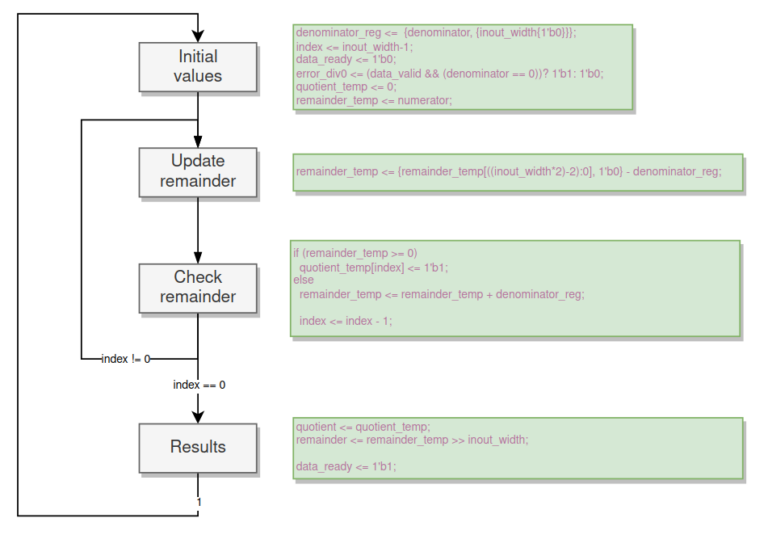Notice that the width of remainder_temp and denominator_reg are twice than the input width. The verilog code of the FSM can be find next.

case (div_status)
2'd0: begin
if (data_valid && (denominator != 0)) div_status <= 2'd1;
else div_status <= 2'd0;

denominator_reg <=  {denominator, {inout_width{1'b0}}};
index <= inout_width-1;
error_div0 <= (data_valid && (denominator == 0))? 1'b1: 1'b0;
quotient_temp <= 0;
remainder_temp <= numerator;
end
2'd1: begin
div_status <= 2'd2;

remainder_temp <= {remainder_temp[((inout_width*2)-2):0], 1'b0} - denominator_reg;
end
2'd2: begin
if (index == 0) div_status <= 2'd3;
else div_status <= 2'd1;

if (remainder_temp >= 0)
quotient_temp[index] <= 1'b1;
else
remainder_temp <= remainder_temp + denominator_reg;

index <= index - 1;
end
2'd3: begin
div_status <= 2'd0;

quotient <= quotient_temp;
remainder <= remainder_temp >> inout_width;

end

endcase


The next algorithm is non restoring division, and as you will find out for its name, this algorithm does not perform a restoration, and this make the algorithm saves one state. In short, this algorithm does not wait to see the sign of the remainder for add or subtract the denominator, it add and subtract according the sign of the last remainder. Then, when the iterations are finished, the quotient has to be prepared according the sign of the last remainder. This have to be done because the original theoretic non-restoring algorithm puts a minus one in the quotient bit when the remainder is less than zero. To fix that, we need to perform the ones complement on the final result. The FSM with the code can be find next.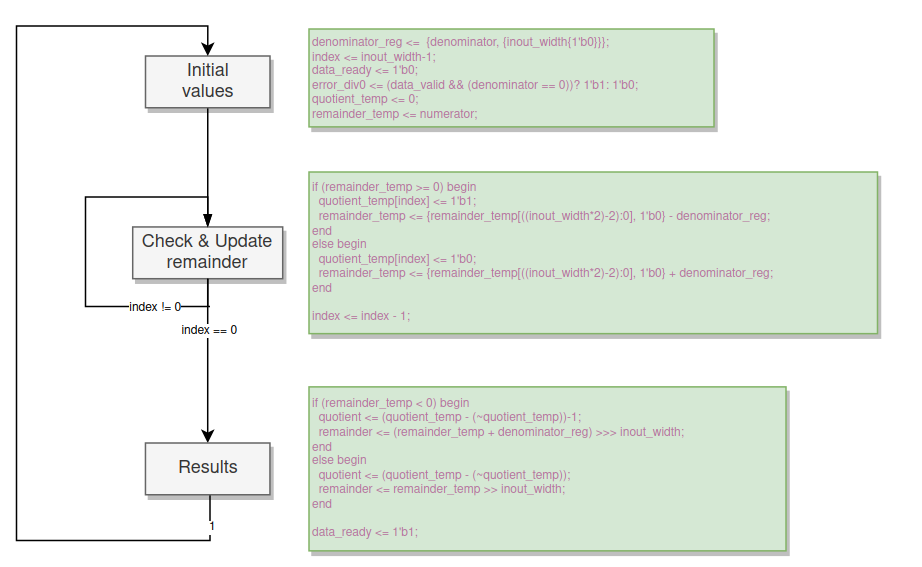You can see that this time, the FSM only have 3 state, so the number of clock cycles spent in the calculation is 2+n.

case (div_status)
2'd0: begin
if (data_valid && (denominator != 0)) div_status <= 2'd1;
else div_status <= 2'd0;

denominator_reg <=  {denominator, {inout_width{1'b0}}};
index <= inout_width-1;
error_div0 <= (data_valid && (denominator == 0))? 1'b1: 1'b0;
quotient_temp <= 0;
remainder_temp <= numerator;
end
2'd1: begin
if (index == 0) div_status <= 2'd2;
else div_status <= 2'd1;

if (remainder_temp >= 0) begin
quotient_temp[index] <= 1'b1;
remainder_temp <= {remainder_temp[((inout_width*2)-2):0], 1'b0} - denominator_reg;
end
else begin
quotient_temp[index] <= 1'b0;
remainder_temp <= {remainder_temp[((inout_width*2)-2):0], 1'b0} + denominator_reg;
end

index <= index - 1;
end
2'd2: begin
div_status <= 2'd0;

if (remainder_temp < 0) begin
quotient <= (quotient_temp - (~quotient_temp))-1;
remainder <= (remainder_temp + denominator_reg) >>> inout_width;
end
else begin
quotient <= (quotient_temp - (~quotient_temp));
remainder <= remainder_temp >> inout_width;
end

end

endcase


Until now, the algorithm we have seen only performs unsigned operations. To add signed support, we have to make some modifications to the code. To obtain a signed quotient, in the first state we have to store the sign of the numerator and the denominator. If the signs are different, the resulting quotient will be negative, in the case that the signs are the same, the resulting quotient will be positive. To detect this differenc in the sign, I have used the operator XOR with the MSb of the numerator and the denominator. Then, I have make positive both numerator and denominator to operate with them as before.

2'd0: begin
if (data_valid && (denominator != 0)) div_status <= 2'd1;
else div_status <= 2'd0;

denominator_reg <=  denominator[inout_width-1]? {-denominator, {inout_width{1'b0}}}: {denominator, {inout_width{1'b0}}};
index <= inout_width-1;
error_div0 <= (data_valid && (denominator == 0))? 1'b1: 1'b0;
quotient_temp <= 0;
remainder_temp <= numerator[inout_width-1]? -numerator: numerator;
sign = denominator[inout_width-1] ^ numerator[inout_width-1];
end


Finally, in the last state, if the sign stored is equal to 1, I have changed the sign of the quotient. Regarding the remainder, since it is not used in the most of the applications, it keeps the same sign always in this case.

2'd2: begin
div_status <= 2'd0;

if (remainder_temp < 0) begin
quotient <= sign? -(quotient_temp - (~quotient_temp))+1: (quotient_temp - (~quotient_temp))-1;
remainder <= (remainder_temp + denominator_reg) >>> inout_width;
end
else begin
quotient <= sign? -(quotient_temp - (~quotient_temp)): (quotient_temp - (~quotient_temp));
remainder <= remainder_temp >> inout_width;
end

end


In the next figure you can see the difference in clock cycles used by the long division algorithm _ld, restoring _r, non-restoring _nr and signed non restoring _snr. As I mentioned before, the time spent by the non restoring is almost the half than the others.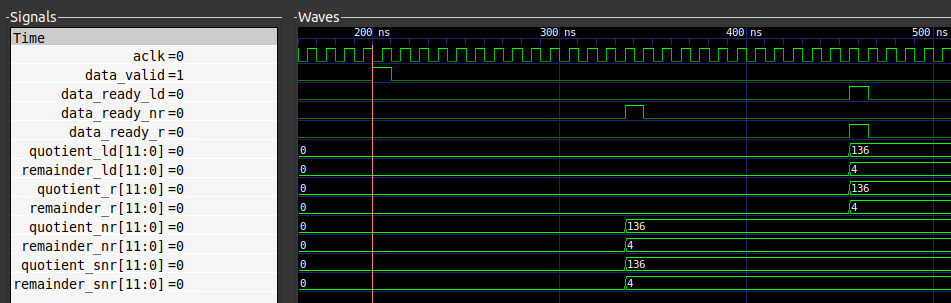Regarding the utilization, we can see that the lightest, for my implementations, is long division, but if we think in terms of utilization / speed ratio, non-restoring is the best choice. Also we can see how adding the sign management increase the number of LUTs due to the comparisons and the sign change.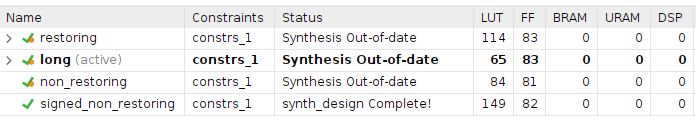There are other methods to perform divisions, even we can calculate divisions using CORDIC algorithm, but it is not direct, since we have to calculate two trigonometric functions, and then obtain the quotient. Other methods uses look-up tables to compute the quotient like the SRT method, named for its creators Sweenie, Robertson and Tocher. This method was the cause of the first Pentium processors failure, due to the look-up table was incorrectly indexed.

Other methods like Newton – Raphson, uses series of approximations to find the reciprocal of the denominator, and then the result is multiplied by the numerator. Newton method is used to find the zeroes of a function, so we can rewrite the reciprocal in order to be equal to zero.

$\frac{1}{D}=x \rightarrow \frac{1}{x}-D=0$

Then the function to make zero will be the next.

$f(x)=\frac{1}{x}-D=0$

To use the Newton – Raphson method we need to find also the derivative of the function.

$f'(x)=\frac{1}{x^2}$

Now, we have to replace the corresponding values in the Newton – Raphson series equation.

$x_{n+1}=x_n-\frac{f(x)}{f'(x)}$ $x_{n+1}=x_n-\frac{\frac{1}{x}-D}{\frac{1}{x^2}}=x_n(2-D \cdot x_n)$

Then, iterating by this equation we can find the reciprocal. Notice that 2 multiplications are needed in each iteration, and also a third multiplication is needed to find the result of the division, since we have to multiply the reciprocal of the denominator by the numerator. The difficult of this method is to find the first value for $$x_n$$, since an incorrect value could make the algorithm diverge to the infinite. There are some method to find a correct initial value. Here you can find a document that talks about it.

For many of digital signal processing algorithms, divisions are not used. We don’t use divisions in filter, neither FIR or IIR, compute DFT or FFT, convolutions… but some times we need to perform a division, for example to compute frequency. In power electronics, divisions are needed to know, before the converter starts, the relation between the voltage in the input of the converter and the voltage in the output of the converter. This relation is the duty cycle that I have to apply when the converter starts switching in order to prevent the current flow. We have seen different methods, but the most interesting for me is the non-restoring. It is simple, does not use any more than logic, and for regular data widths like 12 or 16 bits, the amount of time spent is reasonable. If you need go faster, Newton – Raphson is a good option, since you can obtain a good approximation in 5 or 6 iterations, but you will use 3 multipliers. In any case, the method used will depend of the application.